# Chapter 2 Quickly Exploring Data

Although I’ve used the ggplot2 package for most of the graphics in this book, it is not the only way to plot data. For very quick exploration of data, it’s sometimes useful to use the plotting functions in base R. These are installed by default with R and do not require any additional packages to be installed. They’re quick to type, straightforward to use in simple cases, and run very quickly.

If you want to do anything beyond very simple plots, though, it’s generally better to switch to ggplot2. This is in part because ggplot2 provides a unified interface and set of options, instead of the grab bag of modifiers and special cases required in base graphics. Once you learn how ggplot2 works, you can use that knowledge for everything from scatter plots and histograms to violin plots and maps.

Each recipe in this section shows how to make a graph with base graphics. Each recipe also shows how to make a similar graph with the `ggplot()` function in ggplot2. The previous edition of this book also gave examples using the `qplot()` function from the ggplot2 package, but now it is recommended to just use `ggplot()` instead.

If you already know how to use R’s base graphics, having these examples side by side will help you transition to using ggplot2 for when you want to make more sophisticated graphics.

## 2.1 Creating a Scatter Plot

### 2.1.1 Problem

You want to create a scatter plot.

### 2.1.2 Solution

To make a scatter plot (Figure 2.1), use `plot()` and pass it a vector of x values followed by a vector of y values:

``plot(mtcars\$wt, mtcars\$mpg)``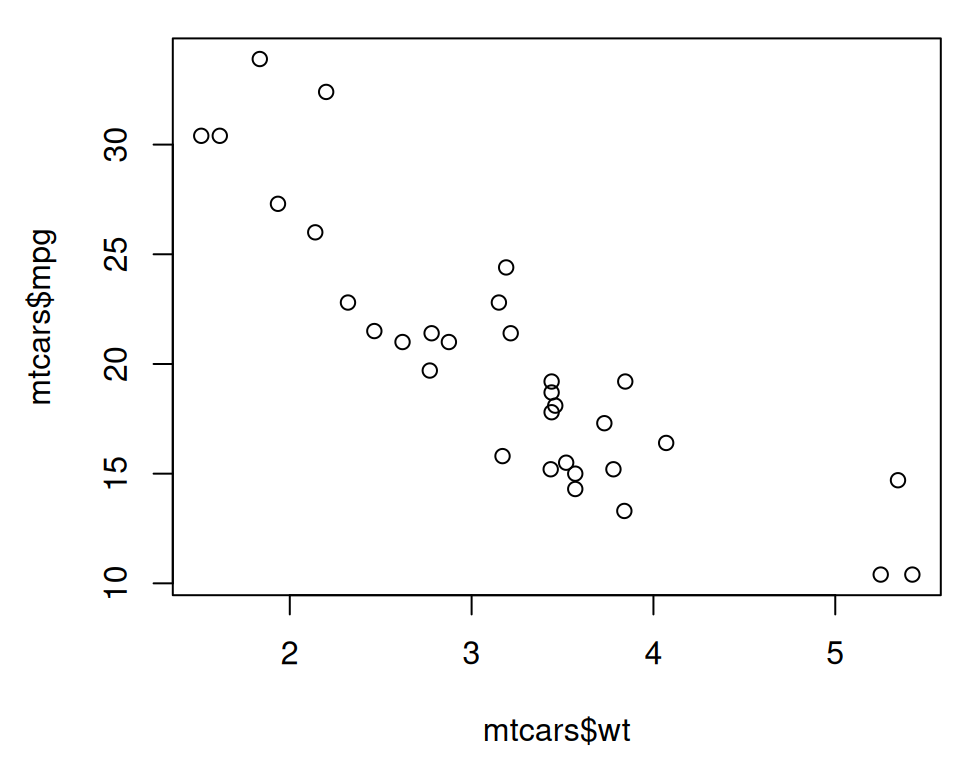Figure 2.1: Scatter plot with base graphics

The `mtcars\$wt` returns the column named `wt` from the `mtcars` data frame, and `mtcars\$mpg` is the `mpg` column.

With ggplot2, you can get a similar result using the `ggplot()` function (Figure Figure 2.2):

``````library(ggplot2)

ggplot(mtcars, aes(x = wt, y = mpg)) +
geom_point()``````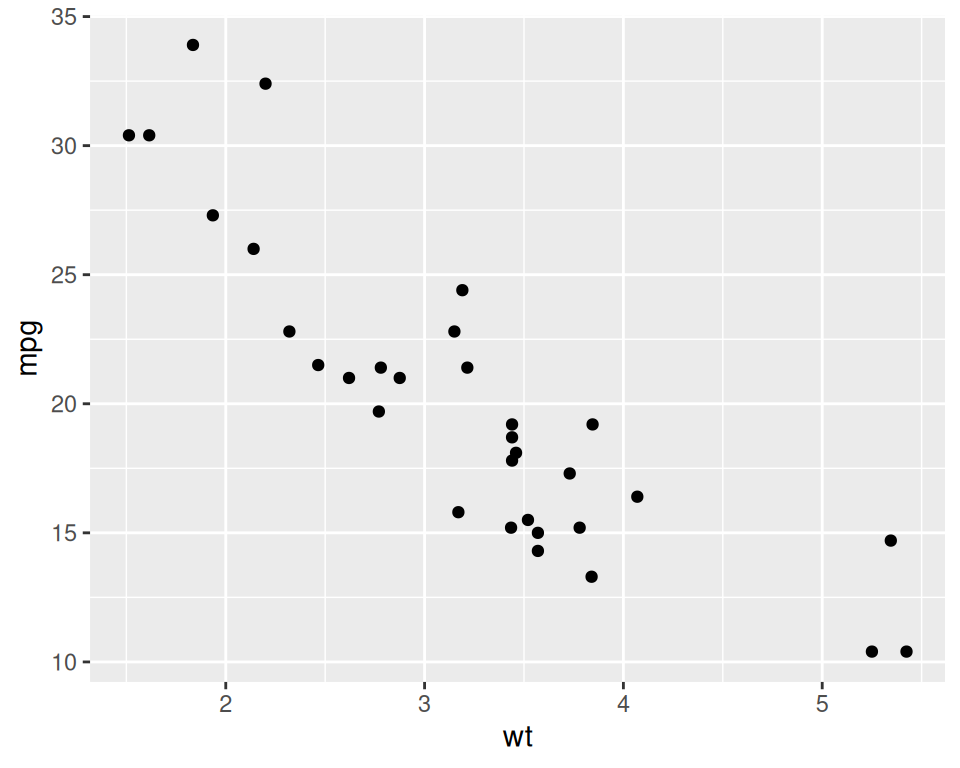Figure 2.2: Scatter plot with ggplot2

The first part, `ggplot()`, tell it to create a plot object, and the second part, `geom_point()`, tells it to add a layer of points to the plot.

The usual way to use `ggplot()` is to pass it a data frame (`mtcars`) and then tell it which columns to use for the x and y values. If you want to pass it two vectors for x and y values, you can use `data = NULL`, and then pass it the vectors. Keep in mind that ggplot2 is designed to work with data frames as the data source, not individual vectors, and that using it this way will only allow you to use a limited part of its capabilities.

``````ggplot(data = NULL, aes(x = mtcars\$wt, y = mtcars\$mpg)) +
geom_point()``````

It is common to see `ggplot()` commands spread across multiple lines, so you may see the above code also written like this:

### 2.1.3 See Also

See Chapter 5 for more in-depth information about creating scatter plots.

## 2.2 Creating a Line Graph

### 2.2.1 Problem

You want to create a line graph.

### 2.2.2 Solution

To make a line graph using `plot()` (Figure 2.3, left), pass it a vector of x values and a vector of y values, and use `type = "l"`:

``plot(pressure\$temperature, pressure\$pressure, type = "l")``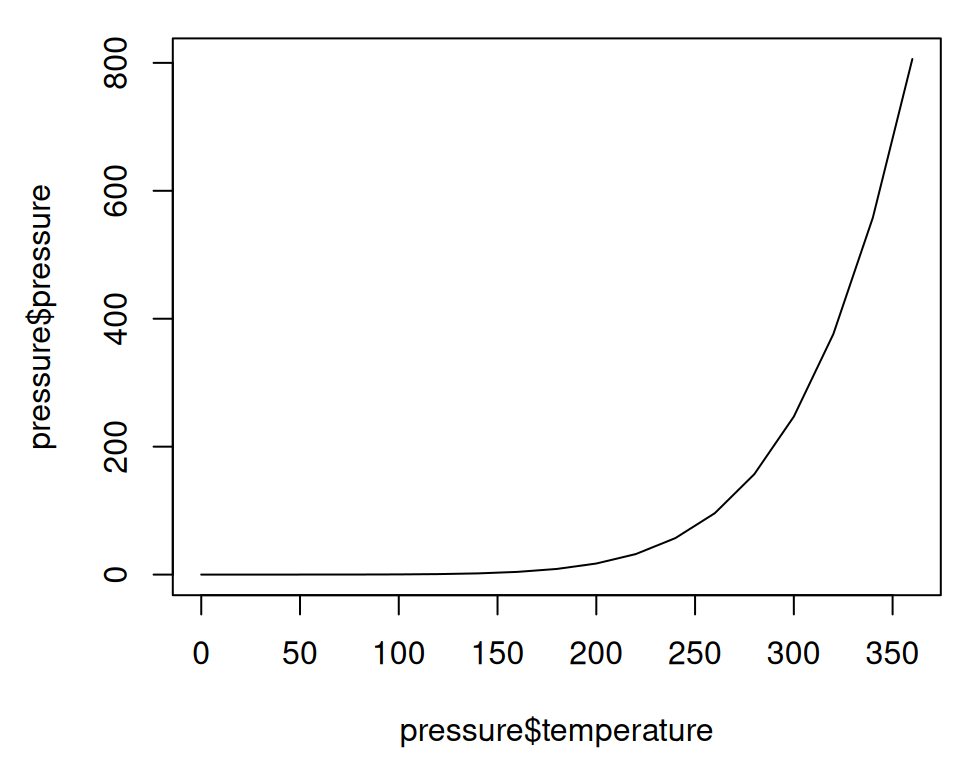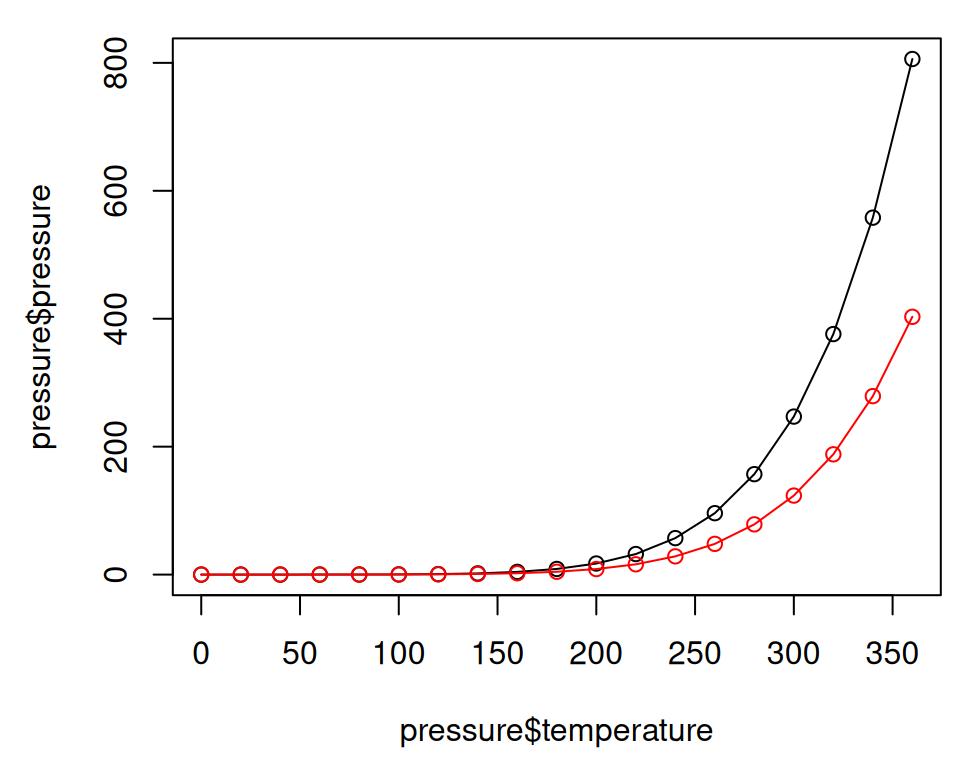Figure 2.3: Line graph with base graphics (left); With points and another line (right)

To add points and/or multiple lines (Figure 2.3, right), first call `plot()` for the first line, then add points with `points()` and additional lines with `lines()`:

``````plot(pressure\$temperature, pressure\$pressure, type = "l")
points(pressure\$temperature, pressure\$pressure)

lines(pressure\$temperature, pressure\$pressure/2, col = "red")
points(pressure\$temperature, pressure\$pressure/2, col = "red")``````

With ggplot2, you can get a similar result using `geom_line()` (Figure 2.4):

``````library(ggplot2)
ggplot(pressure, aes(x = temperature, y = pressure)) +
geom_line()``````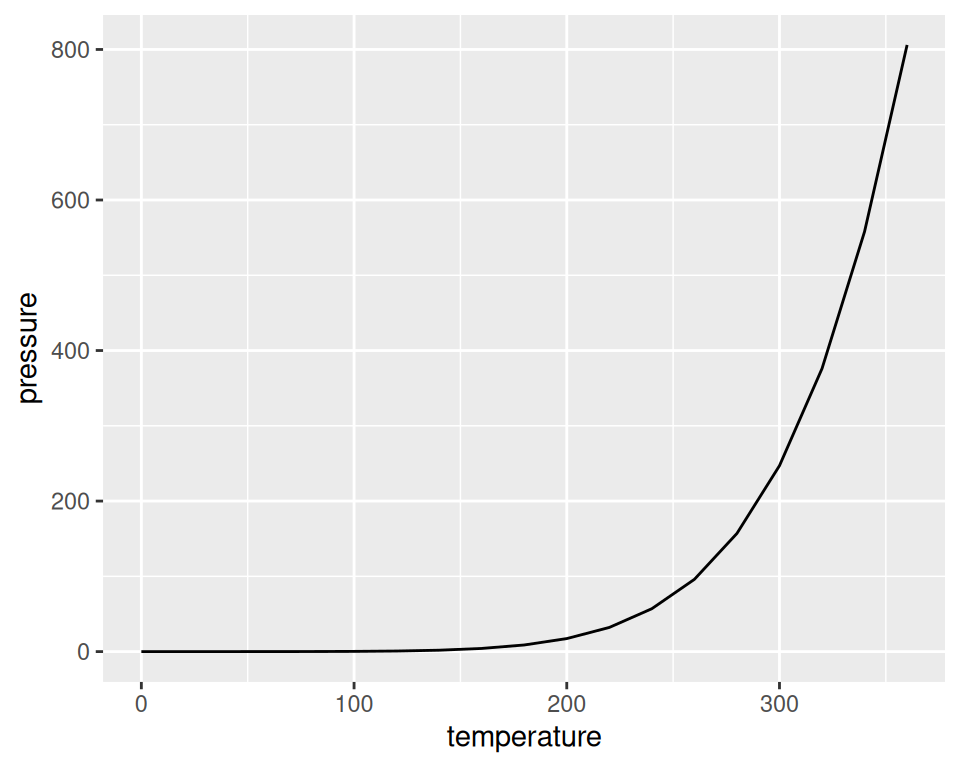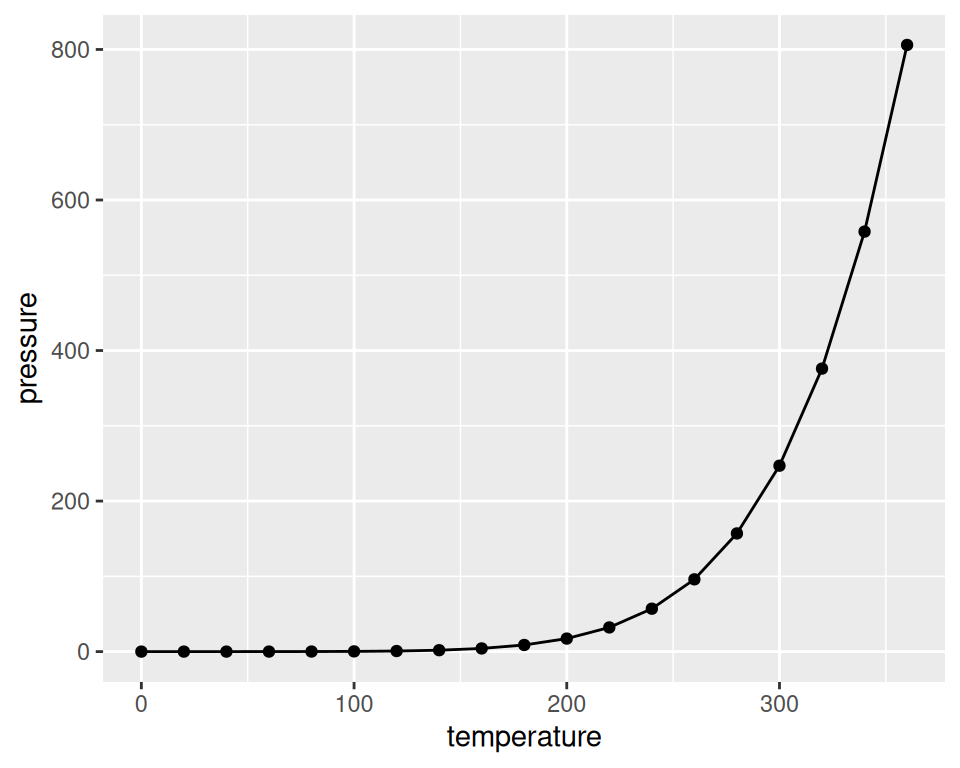Figure 2.4: Line graph with `ggplot()` (left); With points added (right)

As with scatter plots, you can pass you data in vectors instead of in a data frame (but this will limit the things you can do later with the plot):

``````ggplot(pressure, aes(x = temperature, y = pressure)) +
geom_line() +
geom_point()``````

Note

It’s common with `ggplot()` to split the command on multiple lines, ending each line with a `+` so that R knows that the command will continue on the next line.

### 2.2.3 See Also

See Chapter 4 for more in-depth information about creating line graphs.

## 2.3 Creating a Bar Graph

### 2.3.1 Problem

You want to make a bar graph.

### 2.3.2 Solution

To make a bar graph of values (Figure 2.5, left), use `barplot()` and pass it a vector of values for the height of each bar and (optionally) a vector of labels for each bar. If the vector has names for the elements, the names will automatically be used as labels:

``````# First, take a look at the BOD data
BOD
#>   Time demand
#> 1    1    8.3
#> 2    2   10.3
#> 3    3   19.0
#> 4    4   16.0
#> 5    5   15.6
#> 6    7   19.8``````
``barplot(BOD\$demand, names.arg = BOD\$Time)``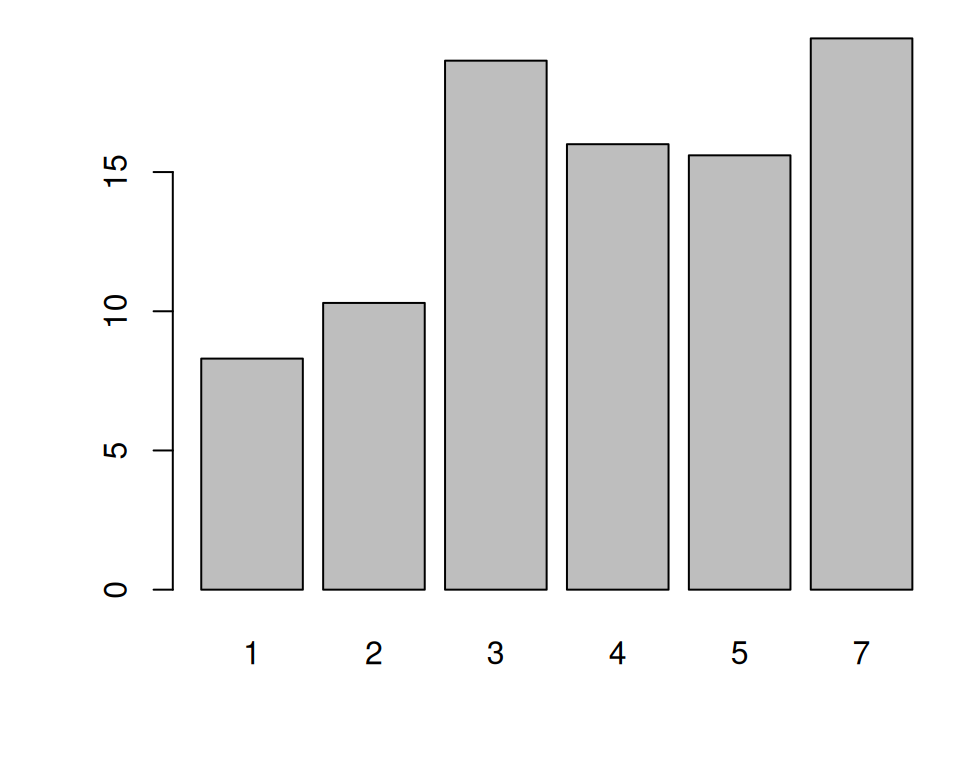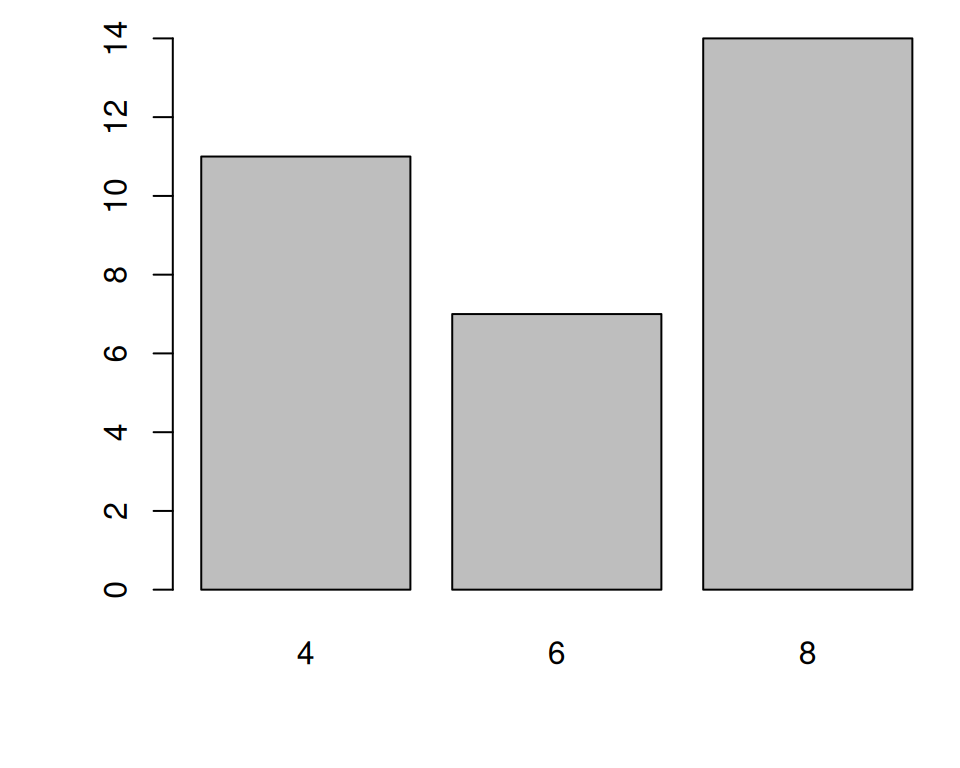Figure 2.5: Bar graph of values with base graphics (left); Bar graph of counts (right)

Sometimes “bar graph” refers to a graph where the bars represent the count of cases in each category. This is similar to a histogram, but with a discrete instead of continuous x-axis. To generate the count of each unique value in a vector, use the `table()` function:

``````# There are 11 cases of the value 4, 7 cases of 6, and 14 cases of 8
table(mtcars\$cyl)``````

Then pass the table to `barplot()` to generate the graph of counts:

``````# Generate a table of counts
barplot(table(mtcars\$cyl))``````

With ggplot2, you can get a similar result using `geom_col()` (Figure 2.6). To plot a bar graph of values, use `geom_col()`. Notice the difference in the output when the x variable is continuous and when it is discrete:

``````library(ggplot2)

# Bar graph of values. This uses the BOD data frame, with the
# "Time" column for x values and the "demand" column for y values.
ggplot(BOD, aes(x = Time, y = demand)) +
geom_col()

# Convert the x variable to a factor, so that it is treated as discrete
ggplot(BOD, aes(x = factor(Time), y = demand)) +
geom_col()``````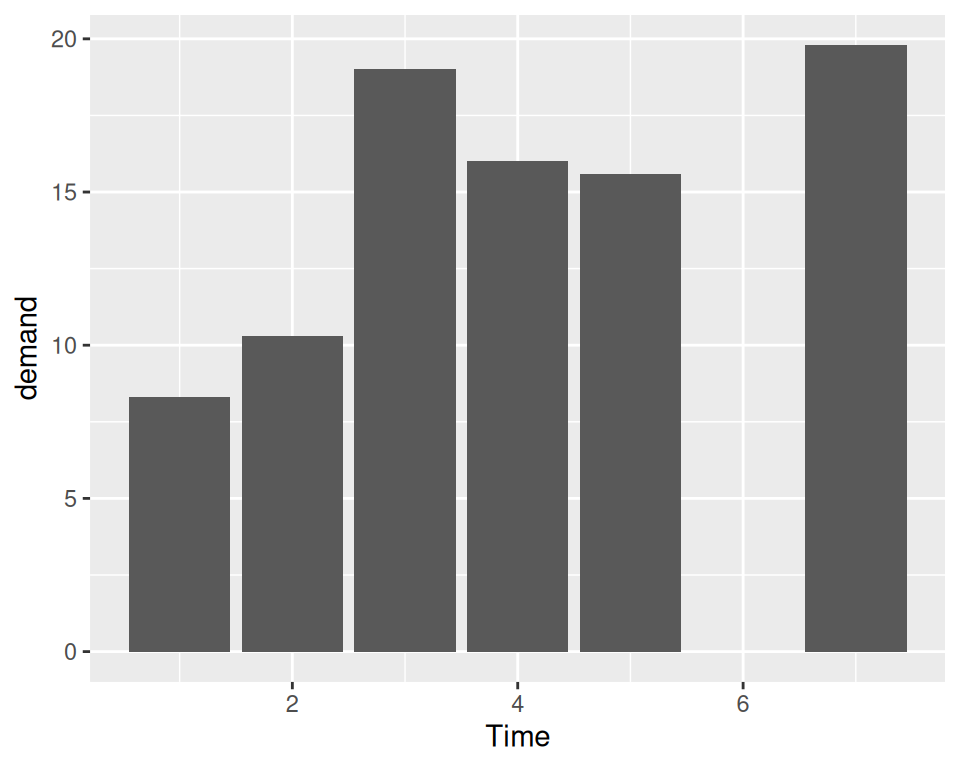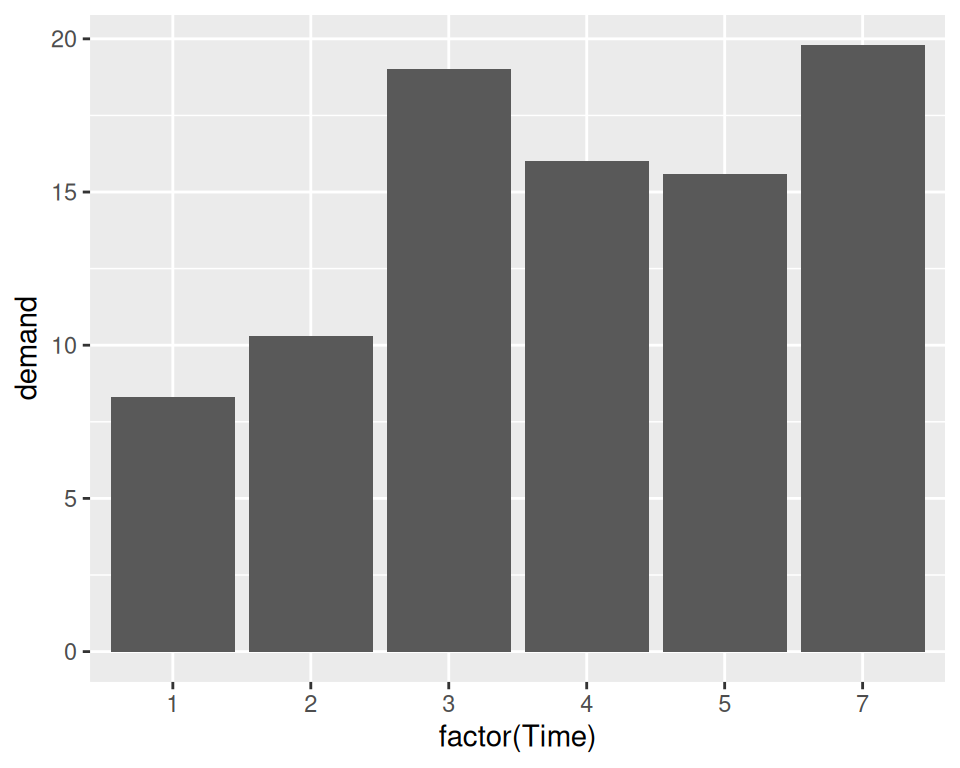Figure 2.6: Bar graph of values using `geom_col()` with a continuous x variable (left); With x variable converted to a factor (notice that there is no entry for 6; right)

ggplot2 can also be used to plot the count of the number of data rows in each category (Figure 2.7, by using `geom_bar()` instead of `geom_col()`. Once again, notice the difference between a continuous x-axis and a discrete one. For some kinds of data, it may make more sense to convert the continuous x variable to a discrete one, with the `factor()` function.

``````# Bar graph of counts This uses the mtcars data frame, with the "cyl" column for
# x position. The y position is calculated by counting the number of rows for
# each value of cyl.
ggplot(mtcars, aes(x = cyl)) +
geom_bar()

# Bar graph of counts
ggplot(mtcars, aes(x = factor(cyl))) +
geom_bar()``````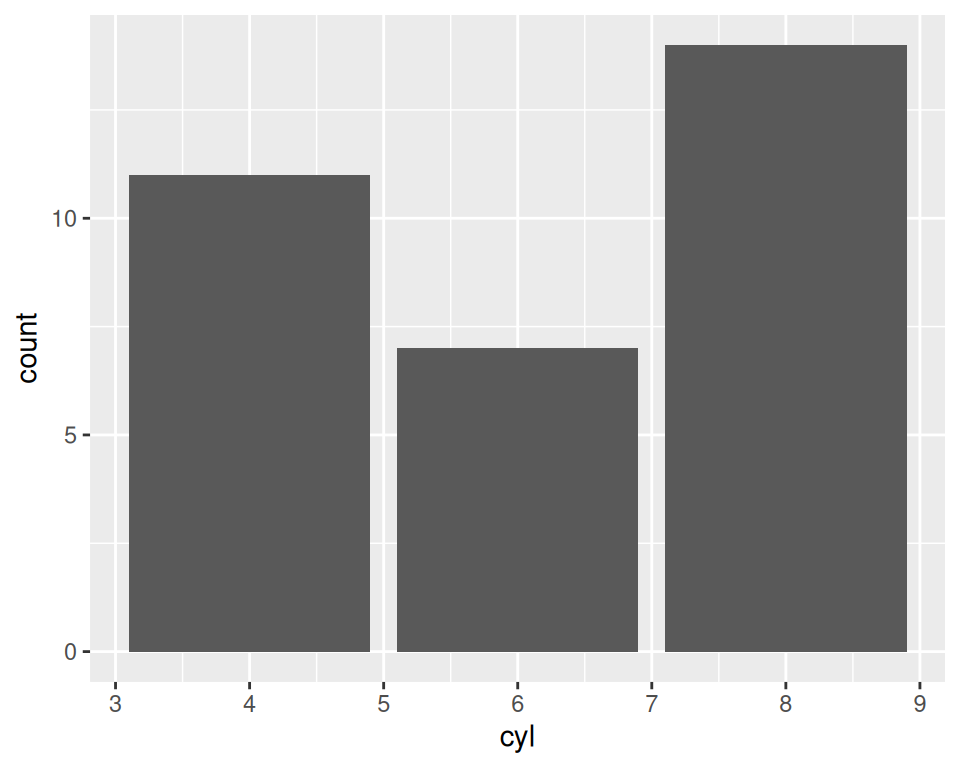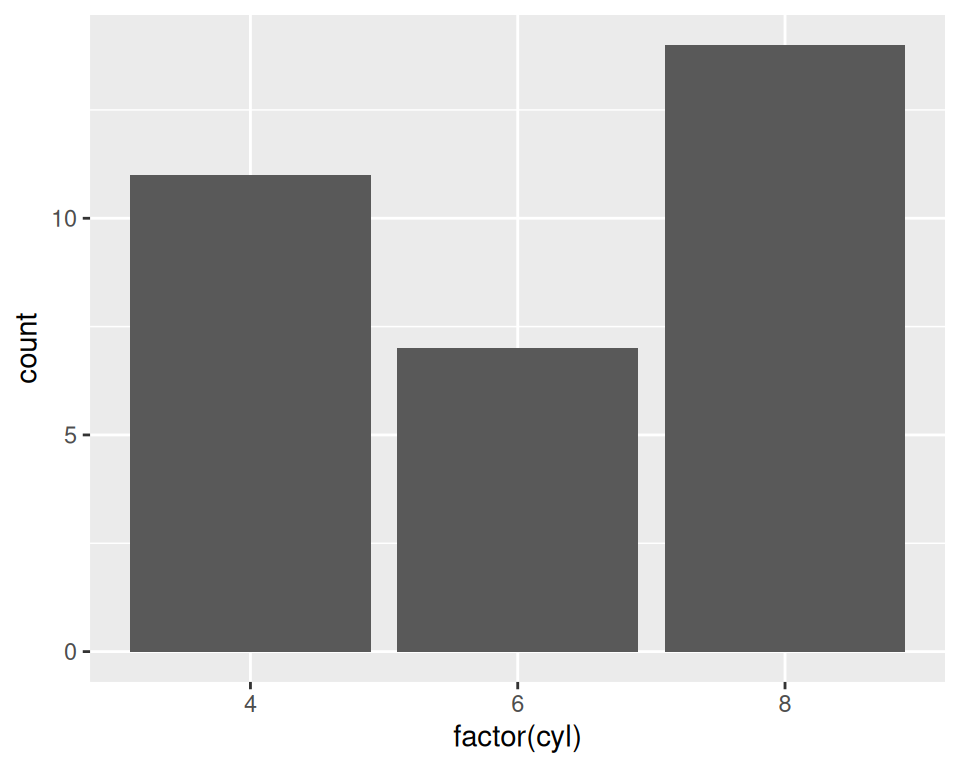Figure 2.7: Bar graph of counts using `geom_bar()` with a continuous x variable (left); With x variable converted to a factor (right)

Note

In previous versions of ggplot2, the recommended way to create a bar graph of values was to use `geom_bar(stat = "identity")`. As of ggplot2 2.2.0, there is a `geom_col()` function which does the same thing.

### 2.3.3 See Also

See Chapter 3 for more in-depth information about creating bar graphs.

## 2.4 Creating a Histogram

### 2.4.1 Problem

You want to view the distribution of one-dimensional data with a histogram.

### 2.4.2 Solution

To make a histogram (Figure 2.8), use `hist()` and pass it a vector of values:

``````hist(mtcars\$mpg)

# Specify approximate number of bins with breaks
hist(mtcars\$mpg, breaks = 10)``````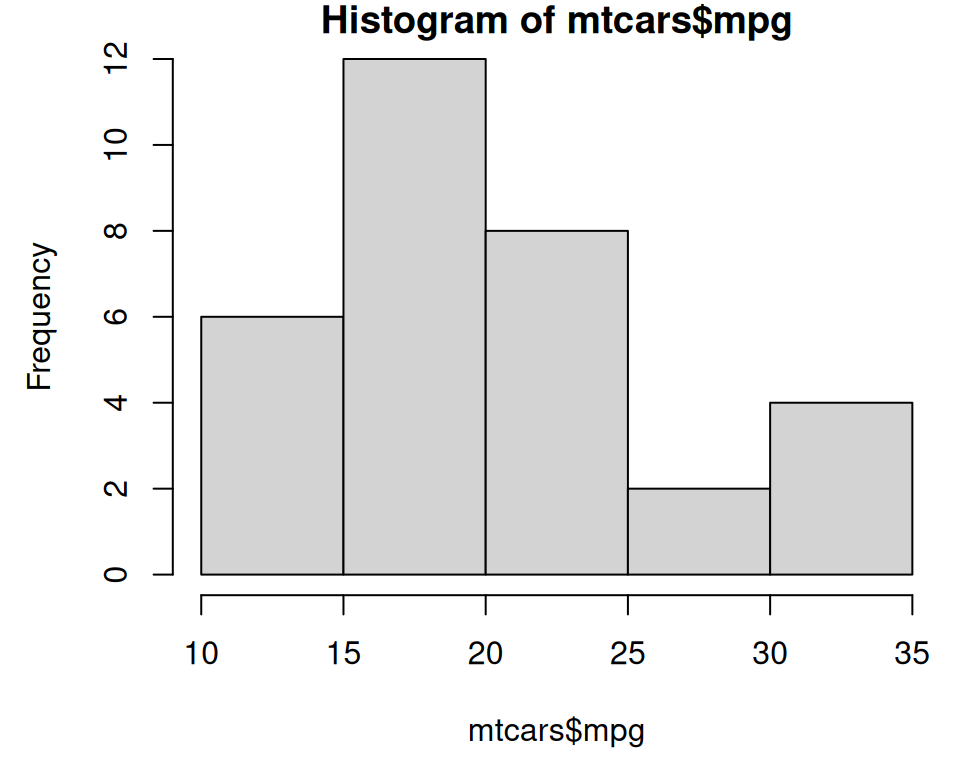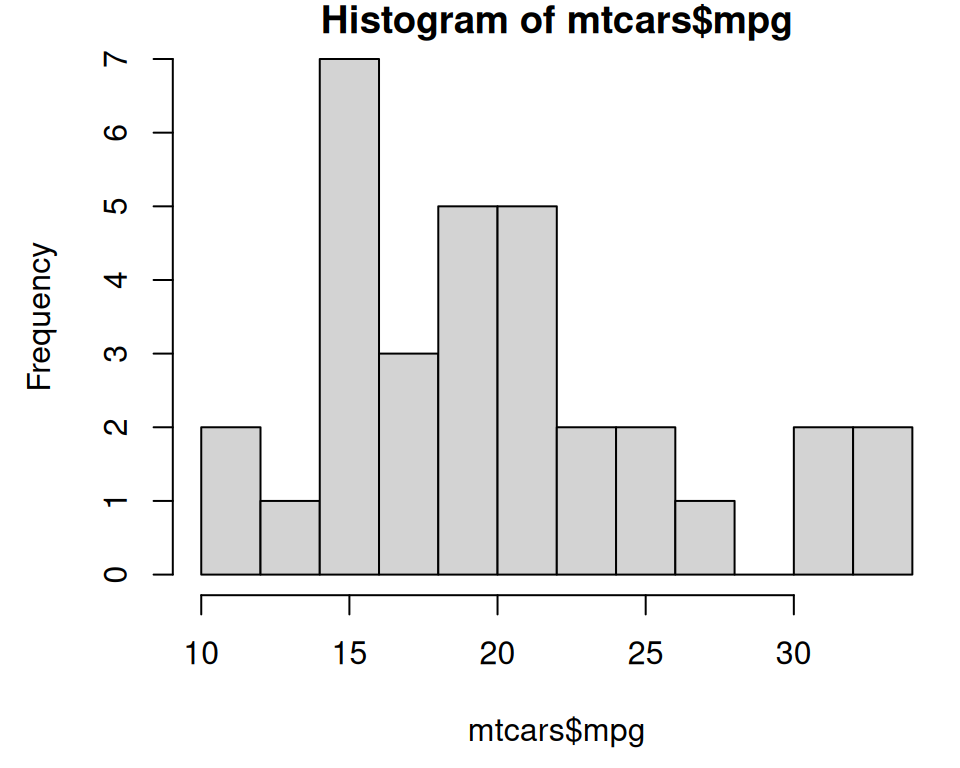Figure 2.8: Histogram with base graphics (left); With more bins. Notice that because the bins are narrower, there are fewer items in each bin. (right)

With the ggplot2, you can get a similar result using `geom_histogram()` (Figure 2.9):

``````library(ggplot2)
ggplot(mtcars, aes(x = mpg)) +
geom_histogram()
#> `stat_bin()` using `bins = 30`. Pick better value with `binwidth`.

# With wider bins
ggplot(mtcars, aes(x = mpg)) +
geom_histogram(binwidth = 4)``````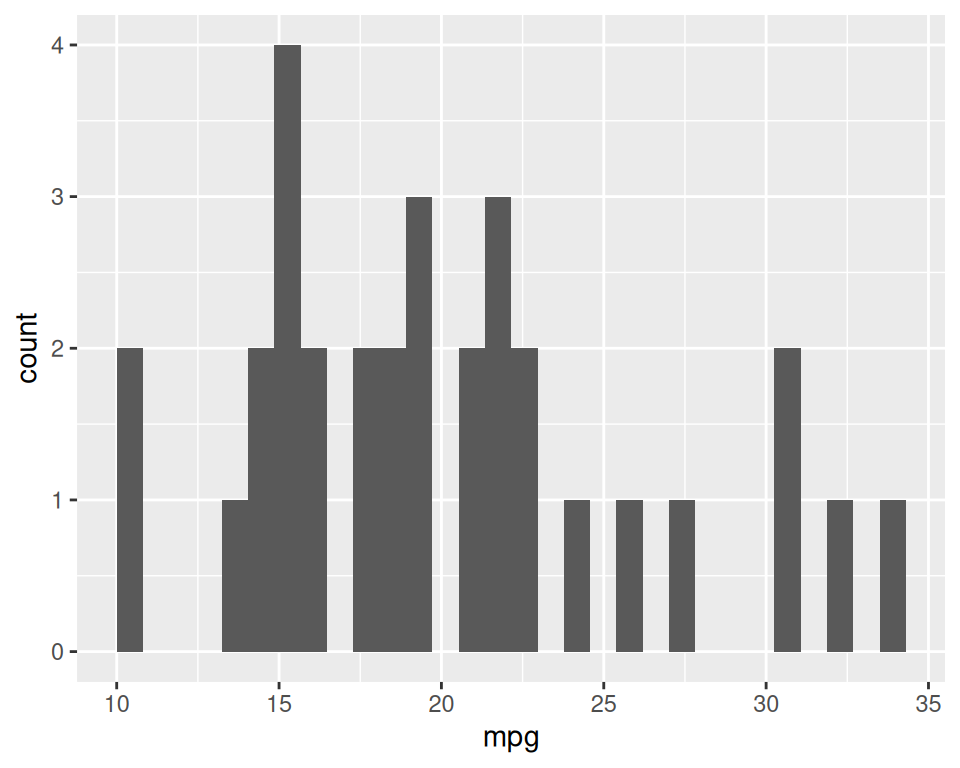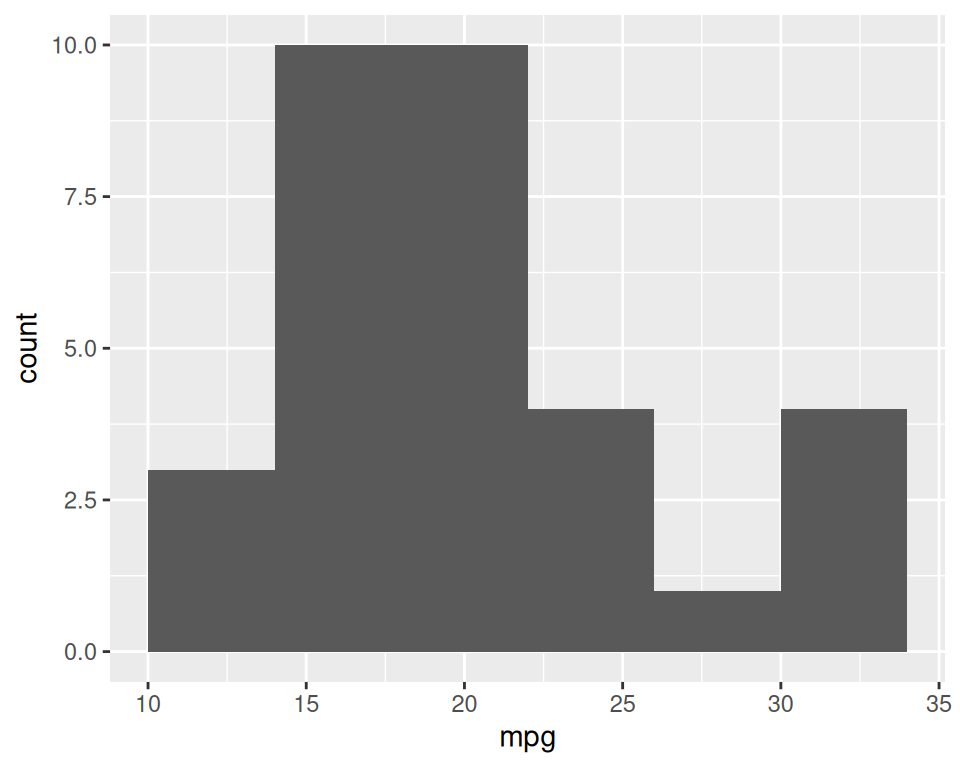Figure 2.9: ggplot2 histogram with default bin width (left); With wider bins (right)

When you create a histogram without specifying the bin width, `ggplot()` prints out a message telling you that it’s defaulting to 30 bins, and to pick a better bin width. This is because it’s important to explore your data using different bin widths; the default of 30 may or may not show you something useful about your data.

### 2.4.3 See Also

For more in-depth information about creating histograms, see Recipes Recipe 6.1 and Recipe 6.2.

## 2.5 Creating a Box Plot

### 2.5.1 Problem

You want to create a box plot for comparing distributions.

### 2.5.2 Solution

To make a box plot (Figure 2.10), use `plot()` and pass it a factor of x values and a vector of y values. When x is a factor (as opposed to a numeric vector), it will automatically create a box plot:

``plot(ToothGrowth\$supp, ToothGrowth\$len)``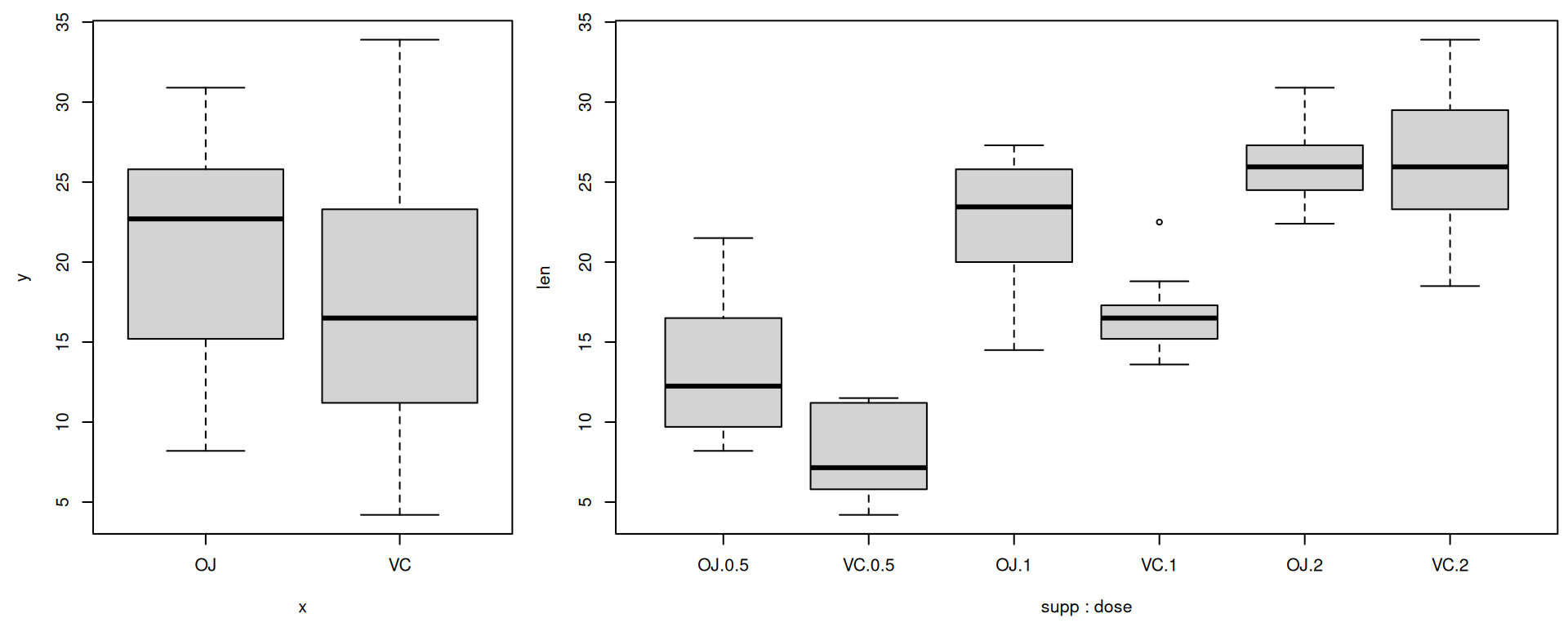Figure 2.10: Box plot with base graphics (left); With multiple grouping variables (right)

If the two vectors are in the same data frame, you can also use the `boxplot()` function with formula syntax. With this syntax, you can combine two variables on the x-axis, as in Figure 2.10:

``````# Formula syntax
boxplot(len ~ supp, data = ToothGrowth)

# Put interaction of two variables on x-axis
boxplot(len ~ supp + dose, data = ToothGrowth)``````

With the ggplot2 package, you can get a similar result (Figure 2.11), with `geom_boxplot()`:

``````library(ggplot2)
ggplot(ToothGrowth, aes(x = supp, y = len)) +
geom_boxplot()``````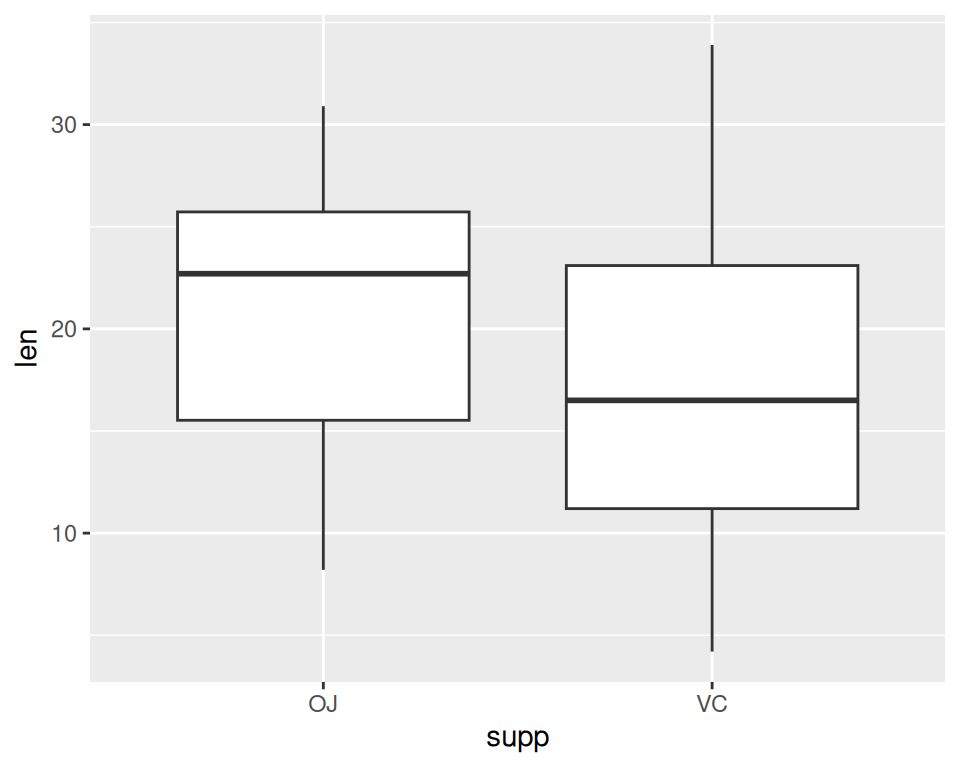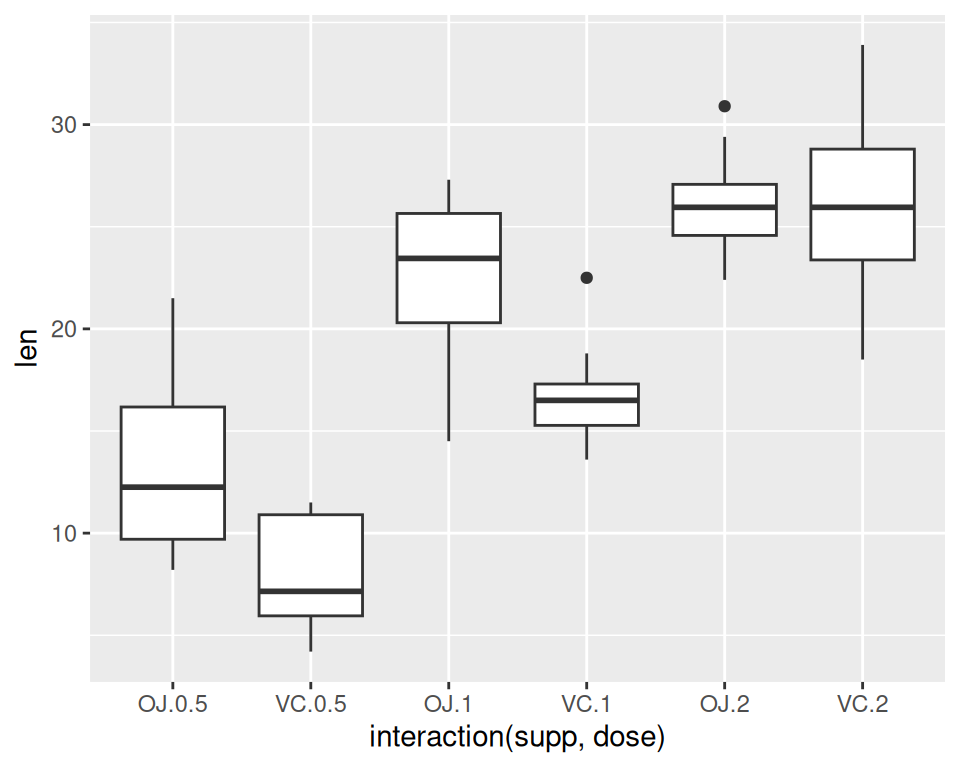Figure 2.11: Box plot with `ggplot()` (left); With multiple grouping variables (right)

It’s also possible to make box plots for multiple variables, by combining the variables with `interaction()`, as in Figure 2.11:

``````ggplot(ToothGrowth, aes(x = interaction(supp, dose), y = len)) +
geom_boxplot()``````

Note

You may have noticed that the box plots from base graphics are ever-so-slightly different from those from ggplot2. This is because they use slightly different methods for calculating quantiles. See `?geom_boxplot` and `?boxplot.stats` for more information on how they differ.

### 2.5.3 See Also

For more on making basic box plots, see Recipe 6.6.

## 2.6 Plotting a Function Curve

### 2.6.1 Problem

You want to plot a function curve.

### 2.6.2 Solution

To plot a function curve, as in Figure 2.12, use `curve()` and pass it an expression with the variable x:

``curve(x^3 - 5*x, from = -4, to = 4)``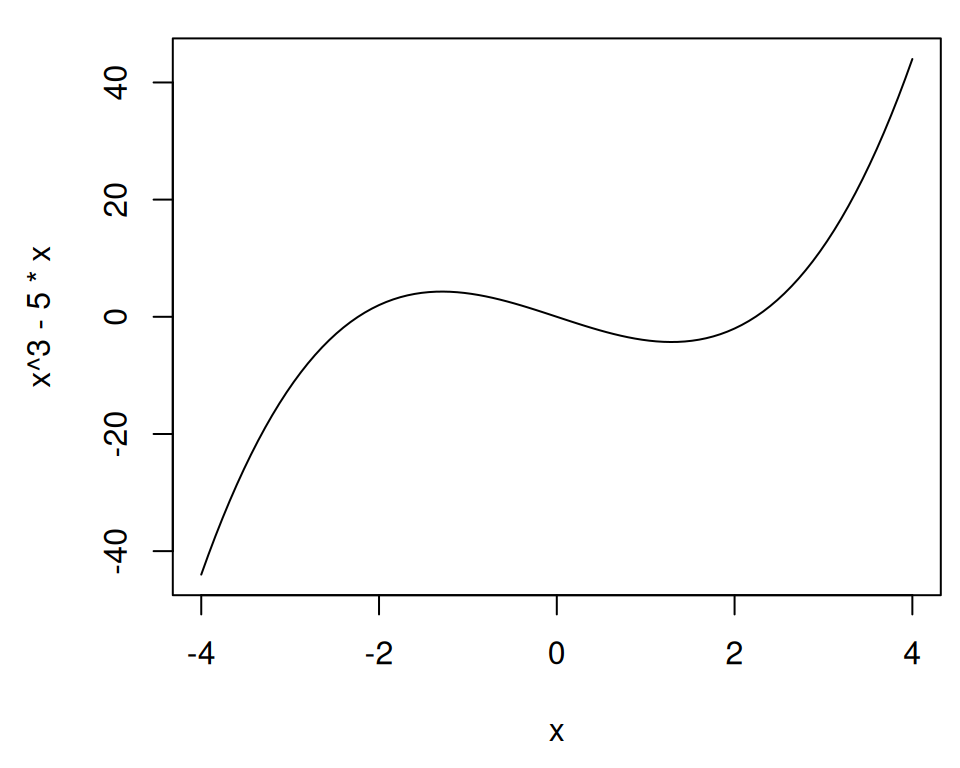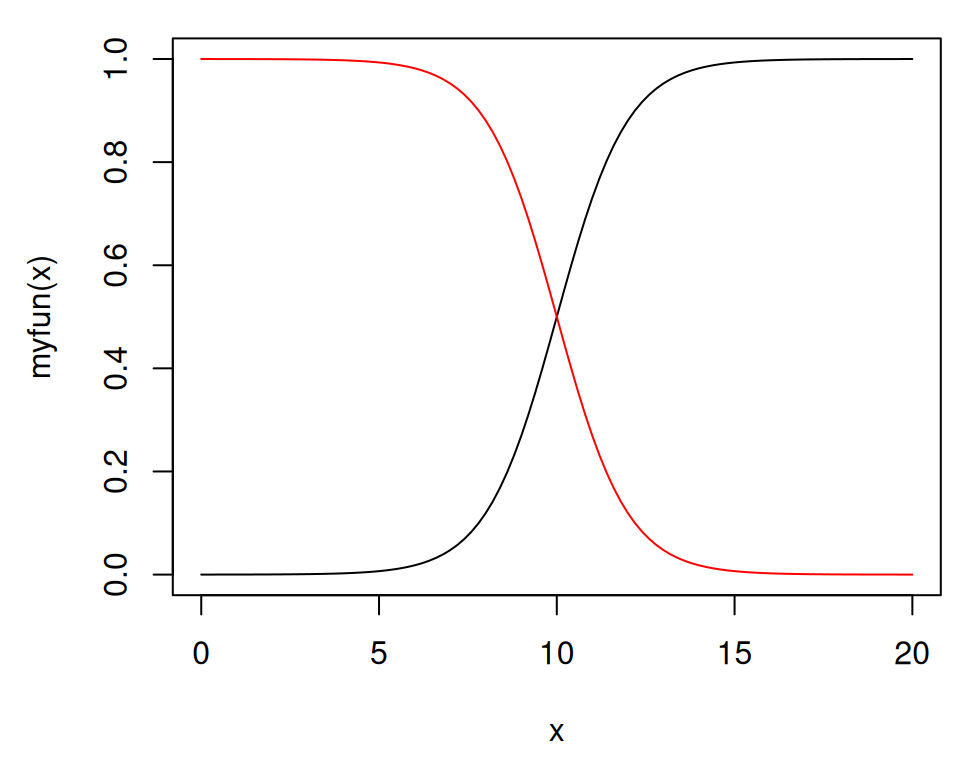Figure 2.12: Function curve with base graphics (left); With user-defined function (right)

You can plot any function that takes a numeric vector as input and returns a numeric vector, including functions that you define yourself. Using `add = TRUE` will add a curve to the previously created plot:

``````# Plot a user-defined function
myfun <- function(xvar) {
1 / (1 + exp(-xvar + 10))
}
curve(myfun(x), from = 0, to = 20)
# Add a line:
curve(1 - myfun(x), add = TRUE, col = "red")``````

With ggplot2, you can get a similar result (Figure 2.13), by using `stat_function(geom = "line")` and passing it a function that takes a numeric vector as input and returns a numeric vector:

``````library(ggplot2)
# This sets the x range from 0 to 20
ggplot(data.frame(x = c(0, 20)), aes(x = x)) +
stat_function(fun = myfun, geom = "line")``````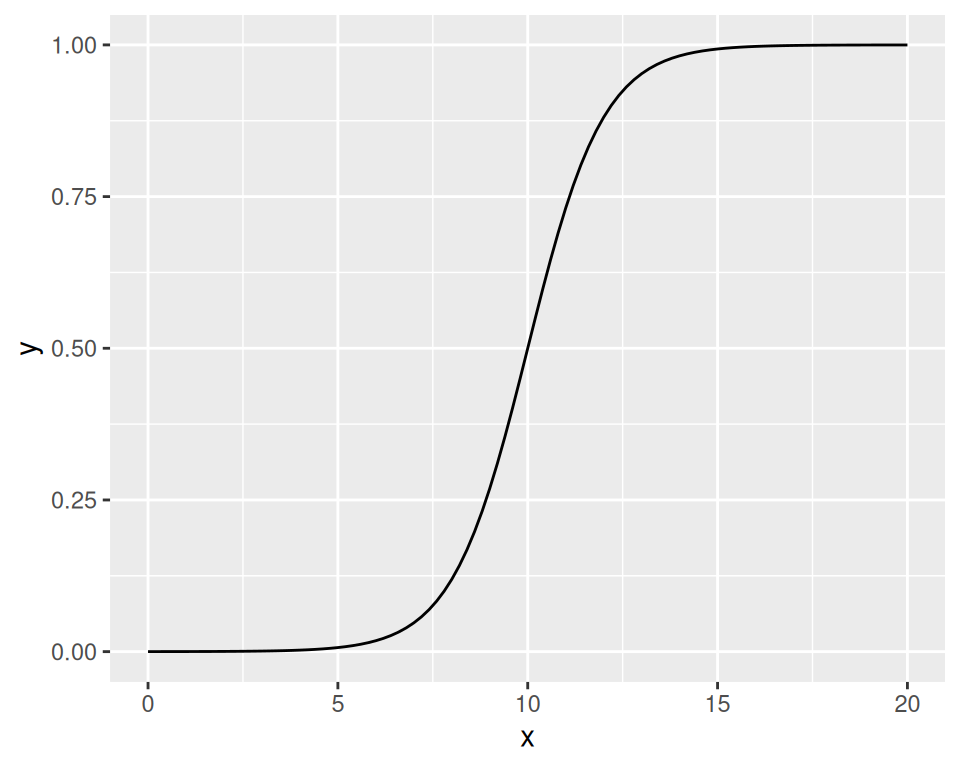Figure 2.13: A function curve with ggplot2

### 2.6.3 See Also

See Recipe 13.2 for more in-depth information about plotting function curves.